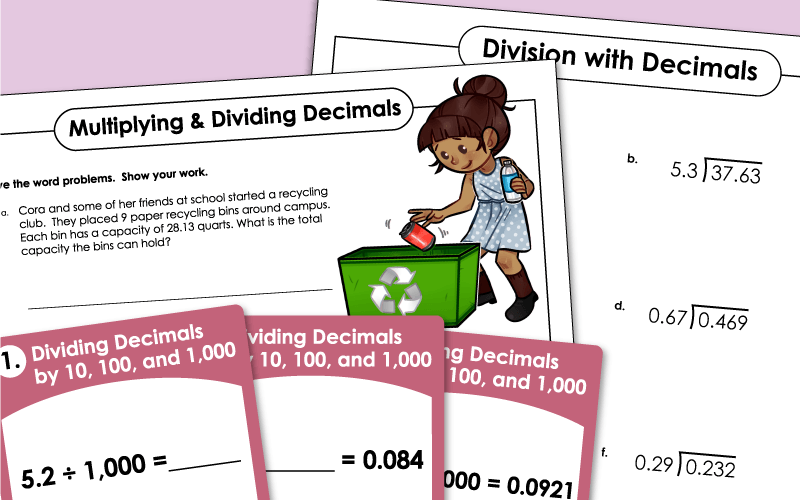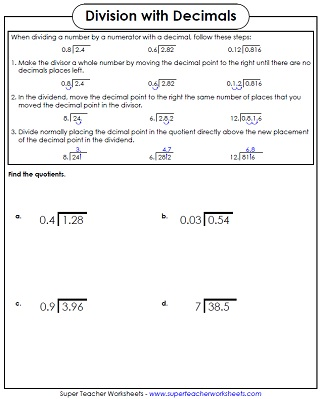# Dividing Decimals

The printable math worksheets on this page can be used to practice dividing decimal numbers.## Decimal Division Patterns

Students will practice dividing decimals by 10, 100, 1,000 and 10,000 with this printable worksheet.
Students will fill in missing dividends, divisors, and quotients on this dividing decimal worksheet.
Practice decimal division patterns with this set of task cards. Divide decimals by 10, 100, and 1,000.

## Decimals in the Dividends(Level: Basic)

This page has five decimal division problems and one word problem. These problems have decimals in the dividend only.
(example: 2.48 divided by 4)
Use these division boxes to teach students how to divide single-digit decimals.
(example: 0.49 divided by 7)
Multiply and divide decimals by single digit numbers to solve the word problems on this worksheet.
Multiply or divide decimals for each word problem on this printable worksheet.

## Decimals in the Divisors and Dividends (Level: Basic)

These problems have decimals in the dividends and divisors. Includes one- and two-digit divisors up to 12. The top of the page explains how to solve.
(example 2.4 divided by 0.8)
These problems have decimals in the divisors and in the dividends. Includes divisors up to 12. Students should be able to complete this page with only a basic knowledge of multiplication, decimals, and long division.
(example: 0.65 divided by 0.5)

## Decimals in theDivisors and Dividends(Level: Intermediate)

These problems have decimals in the divisors and dividends. Includes two-digit divisors. The top of the page explains how to solve.
(example 5.04 divided by 0.42)
These problems have decimals in all parts of the problem.
(example: 77.44 divided by 6.4)
This math worksheet helps students learn how to divide decimals. Turn horizontal math problems into vertical ones and solve. Decimals are in the divisors and dividends.

## Decimals in the Divisor and Dividend (Level: Advanced)

These problems have decimals in the dividends and divisors. Includes two-, three-, and four-digit divisors. Step by step instructions are included on how to solve.
(example 206.01 divided by 7.63)
More advanced-level long division problems with decimals.
(example: 54.288 / 6.24)

Practice adding and subtracting decimal numbers with these printable worksheets.

Multiplying Decimals

Practice solving multiplication problems with decimals as factors.

Decimals (Basic)

Learn to read, write, and interpret basic decimal numbers with these printable worksheets.

## Sample Worksheet ImagesMy Account
Site Information